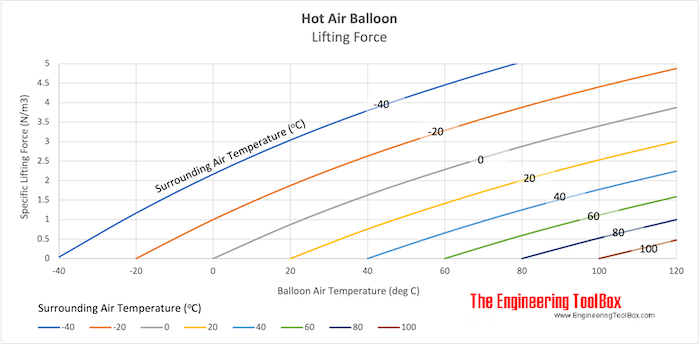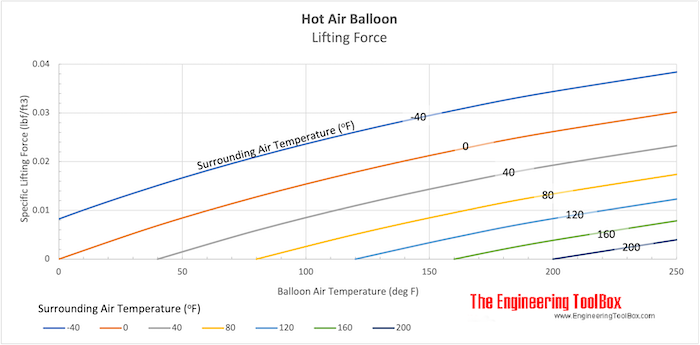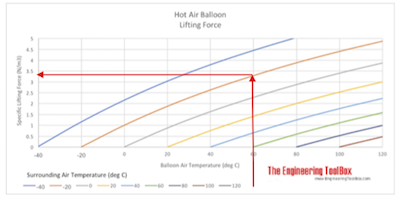Engineering ToolBox - Resources, Tools and Basic Information for Engineering and Design of Technical Applications!

# Hot Air Balloons - Calculate Lifting Weights

## Calculate hot air ballons lifting forces.

### Hot Air Lifting Force

The lifting force from a hot air balloon depends on the density difference between balloon air and surrounding air, and the balloon volume. The lifting force can be calculated as

Fl = V (ρc - ρh) ag                         (1)

where

Fl = lifting force (N, lbf)

V = balloon volume (m3, ft3)

ρc = density cold surrounding air (kg/m3, slugs/ft3)

ρh = density hot balloon air (kg/m3, slugs/ft3)

ag = acceleration of gravity (9.81 m/s2, 32.174 ft/s2)

#### Example - Lifting Force created by a Hot Air Balloon

A hot air balloon with volume 10 m3 (353 ft3) is heated to 100 oC (212 oF).   The temperature of the surrounding air is 20 oC (68 oF). The air density at temperature 100 oC is 0.946 kg/m3 (0.00184 slugs/ft3) and the air density at temperature 20 oC is 1.205 kg/m3 (0.00234 slugs/ft3).

The lifting force can be calculated as

Fl = (10 m3) [(1.205 kg/m3) - (0.946 kg/m3)] (9.81 m/s2)

= 25.4 N

Weight - or gravity force - can be calculated as

Fg = m ag                       (2)

where

Fg = weight - gravity force (N, lbf)

m = mass (kg, slugs)

Since lifting force of a flying air balloon equals weight (Fl = Fg) - the lifted mass can be expressed by combining (1) and (2) to

m = Fl / ag

= (25.4 N) / (9.81 m/s2)

= 2.6 kg

The calculation of lifting force can be done in Imperial units as

Fl = (353 ft3) [(0.00234 slugs/ft3) - (0.00184 slugs/ft3)] (32.174 ft/s2)

= 5.7 lbf

### Hot Air Balloon - Specific Lifting Force

Specific lifting force (force per unit air volume) created by an hot air balloon - balloon temperature vs. surrounded air temperature - are indicated in the charts below.

#### SI - units#### Imperial Units#### Example - Specific Lifting Force from a Hot Air BalloonIf the balloon temperature is 60 oC and the surrounded temperature is -20 oC - the chart indicates a specific lifting force

3.3 N/m3

### Hot Air Balloon Lifting Force Calculator

This calculator can be used to calculate the lifting force of a volume with lower density than surrounding air.

balloon volume (m3)

balloon volume density (kg/m3)

surrounding air density (kg/m3)

## Related Topics

• ### Mechanics

Forces, acceleration, displacement, vectors, motion, momentum, energy of objects and more.

## Related Documents

• ### Air - Thermophysical Properties

Thermal properties of air at different temperatures - density, viscosity, critical temperature and pressure, triple point, enthalpi and entropi, thermal conductivity and diffusivity and more.
• ### Buoyancy

Buoyancy is the resultant force acting on a submerged body.
• ### Elevators - Force and Power

Required force and power to lift an elevator.
• ### Force

Newton's third law - force vs. mass and acceleration.
• ### Lift and Drag

Lift and drag forces acting on a body like an air foil in a fluid flow.
• ### Mass vs. Weight

Mass vs. weight - the Gravity Force.

## Engineering ToolBox - SketchUp Extension - Online 3D modeling!

Add standard and customized parametric components - like flange beams, lumbers, piping, stairs and more - to your Sketchup model with the Engineering ToolBox - SketchUp Extension - enabled for use with older versions of the amazing SketchUp Make and the newer "up to date" SketchUp Pro . Add the Engineering ToolBox extension to your SketchUp Make/Pro from the Extension Warehouse !

We don't collect information from our users. More about

## Citation

• The Engineering ToolBox (2003). Hot Air Balloons - Calculate Lifting Weights. [online] Available at: https://www.engineeringtoolbox.com/hot-air-balloon-lifting-force-d_562.html [Accessed Day Month Year].

Modify the access date according your visit.

9.19.12